1592229900

Model Complexity, Accuracy and Interpretability

Introduction
Complex Real-world challenges requires complex models to be build to give out predictions with utmost accuracy. However, they do not end up being highly interpretable. In this article, we will be looking into the relationship between complexity, accuracy and interpretability.
Motivation:
I am currently working as a Machine Learning Researcher and working on a project on Interpretable Machine Learning. This series is based of my implementation of the book by Christopher Molnar: “Interpretable Machine Learning”.

#model-interpretability #regression #data-science #machine-learning #python

Buddha Community1596191340

Uncovering the Magic: interpreting Machine Learning black-box models

**The trade-off between predictive power and interpretability **is a common issue to face when working with black-box models, especially in business environments where results have to be explained to non-technical audiences. Interpretability is crucial to being able to question, understand, and trust AI and ML systems. It also provides data scientists and engineers better means for debugging models and ensuring that they are working as intended.Motivation

This tutorial aims to present different techniques for approaching model interpretation in black-box models.

_Disclaimer: _this article seeks to introduce some useful techniques from the field of interpretable machine learning to the average data scientists and to motivate its adoption . Most of them have been summarized from this highly recommendable book from Christoph Molnar: Interpretable Machine Learning.

The entire code used in this article can be found in my GitHub

Contents

1. Taxonomy of Interpretability Methods
2. Dataset and Model Training
3. Global Importance
4. Local Importance

1. Taxonomy of Interpretability Methods

• **Intrinsic or Post-Hoc? **This criteria distinguishes whether interpretability is achieved by restricting the complexity of the machine learning model (intrinsic) or by applying methods that analyze the model after training (post-hoc).
• **Model-Specific or Model-Agnostic? **Linear models have a model-specific interpretation, since the interpretation of the regression weights are specific to that sort of models. Similarly, decision trees splits have their own specific interpretation. Model-agnostic tools, on the other hand, can be used on any machine learning model and are applied after the model has been trained (post-hoc).
• **Local or Global? **Local interpretability refers to explaining an individual prediction, whereas global interpretability is related to explaining the model general behavior in the prediction task. Both types of interpretations are important and there are different tools for addressing each of them.

2. Dataset and Model Training

Since the focus of this article is not centered in the modelling phase of the ML pipeline, minimum feature engineering was performed in order to model the data with an XGBoost.

The performance metrics obtained for the model are the following: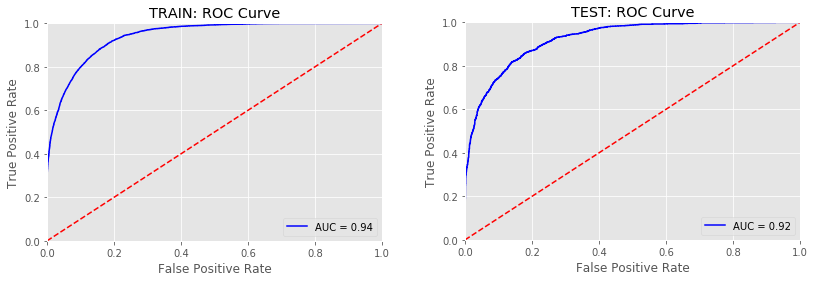Fig. 1: Receiving Operating Characteristic (ROC) curves for Train and Test sets.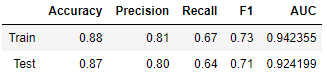Fig. 2: XGBoost performance metrics

The model’s performance seems to be pretty acceptable.

3. Global Importance

The techniques used to evaluate the global behavior of the model will be:

3.1 - Feature Importance (evaluated by the XGBoost model and by SHAP)

3.2 - Summary Plot (SHAP)

3.3 - Permutation Importance (ELI5)

3.4 - Partial Dependence Plot (PDPBox and SHAP)

3.5 - Global Surrogate Model (Decision Tree and Logistic Regression)

3.1 - Feature Importance

• XGBoost (model-specific)
feat_importances = pd.Series(clf_xgb_df.feature_importances_, index=X_train.columns).sort_values(ascending=True)
feat_importances.tail(20).plot(kind='barh')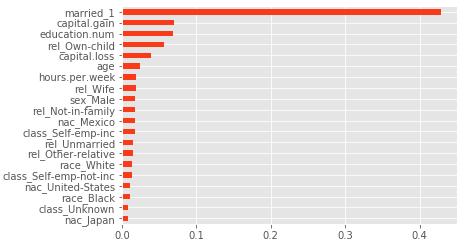Fig. 3: XGBoost Feature Importance

When working with XGBoost, one must be careful when interpreting features importances, since the results might be misleading. This is because the model calculates several importance metrics, with different interpretations. It creates an importance matrix, which is a table with the first column including the names of all the features actually used in the boosted trees, and the other with the resulting ‘importance’ values calculated with different metrics (Gain, Cover, Frequence). A more thourough explanation of these can be found here.

The **Gain **is the most relevant attribute to interpret the relative importance (i.e. improvement in accuracy) of each feature.

• SHAP

In general, SHAP library is considered to be a model-agnostic tool for addressing interpretability (we will cover SHAP’s intuition in the Local Importance section). However, the library has a model-specific method for tree-based machine learning models such as decision trees, random forests and gradient boosted trees.

explainer = shap.TreeExplainer(clf_xgb_df)
shap_values = explainer.shap_values(X_test)

shap.summary_plot(shap_values, X_test, plot_type = 'bar')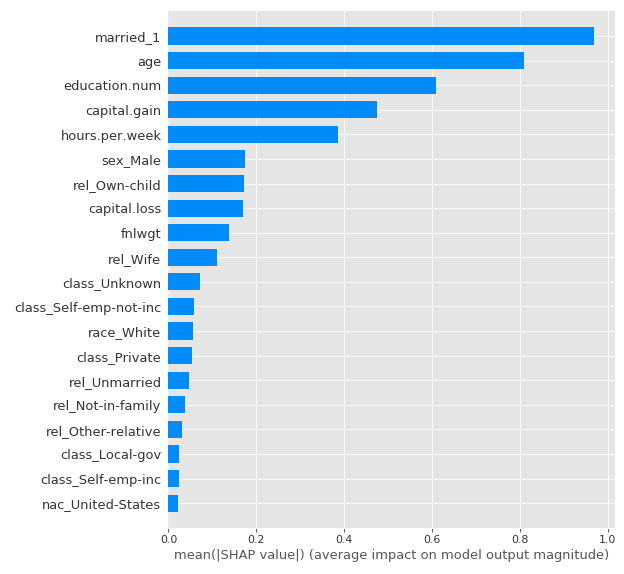Fig. 4: SHAP Feature Importance

The XGBoost feature importance was used to evaluate the relevance of the predictors in the model’s outputs for the Train dataset and the SHAP one to evaluate it for Test dataset, in order to assess if the most important features were similar in both approaches and sets.

It is observed that the most important variables of the model are maintained, although in different order of importance (age seems to take much more relevance in the test set by SHAP approach).

3.2 Summary Plot (SHAP)

The SHAP Summary Plot is a very interesting plot to evaluate the features of the model, since it provides more information than the traditional Feature Importance:

• Feature Importance: variables are sorted in descending order of importance.
• Impact on Prediction: the position on the horizontal axis indicates whether the values of the dataset instances for each feature have more or less impact on the output of the model.
• Original Value: the color indicates, for each feature, whether it is a high or low value (in the range of each of the feature).
• Correlation: the correlation of a feature with the model output can be analyzed by evaluating its color (its range of values) and the impact on the horizontal axis. For example, it is observed that the age has a positive correlation with the target, since the impact on the output increases as the value of the feature increases.
shap.summary_plot(shap_values, X_test)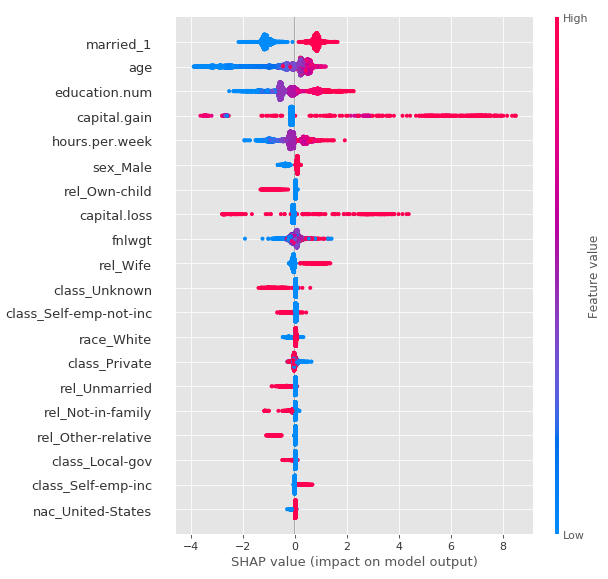Fig. 5: SHAP Summary Plot

3.3 - Permutation Importance (ELI5)

Another way to assess the global importance of the predictors is to randomly permute the order of the instances for each feature in the dataset and predict with the trained model. If by doing this disturbance in the order, the evaluation metric does not change substantially, then the feature is not so relevant. If instead the evaluation metric is affected, then the feature is considered important in the model. This process is done individually for each feature.

To evaluate the trained XGBoost model, the Area Under the Curve (AUC) of the ROC Curve will be used as the performance metric. Permutation Importance will be analyzed in both Train and Test:

# Train
perm = PermutationImportance(clf_xgb_df, scoring = 'roc_auc', random_state=1984).fit(X_train, y_train)
eli5.show_weights(perm, feature_names = X_train.columns.tolist())

# Test
perm = PermutationImportance(clf_xgb_df, scoring = 'roc_auc', random_state=1984).fit(X_test, y_test)
eli5.show_weights(perm, feature_names = X_test.columns.tolist())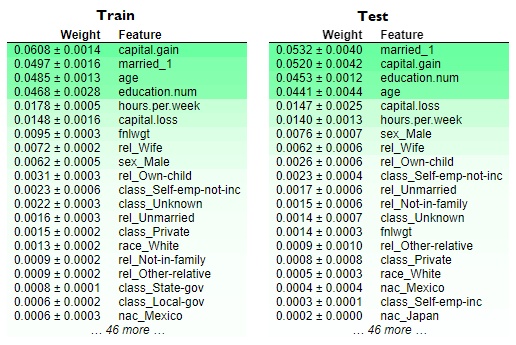Fig. 6: Permutation Importance for Train and Test sets.

Even though the order of the most important features changes, it looks like that the most relevant ones remain the same. It is interesting to note that, unlike the XGBoost Feature Importance, the age variable in the Train set has a fairly strong effect (as showed by SHAP Feature Importance in the Test set). Furthermore, the 6 most important variables according to the Permutation Importance are kept in Train and Test (the difference in order may be due to the distribution of each sample).

The coherence between the different approaches to approximate the global importance generates more confidence in the interpretation of the model’s output.

#model-interpretability #model-fairness #interpretability #machine-learning #shapley-values #deep learning1592257440

Model Complexity, Accuracy and Interpretability

Introduction
Complex Real-world challenges requires complex models to be build to give out predictions with utmost accuracy. However, they do not end up being highly interpretable. In this article, we will be looking into the relationship between complexity, accuracy and interpretability.
Motivation:
I am currently working as a Machine Learning Researcher and working on a project on Interpretable Machine Learning. This series is based of my implementation of the book by Christopher Molnar: “Interpretable Machine Learning”.

#model-interpretability #regression #data-science #machine-learning #python1592229900

Model Complexity, Accuracy and Interpretability

Introduction
Complex Real-world challenges requires complex models to be build to give out predictions with utmost accuracy. However, they do not end up being highly interpretable. In this article, we will be looking into the relationship between complexity, accuracy and interpretability.
Motivation:
I am currently working as a Machine Learning Researcher and working on a project on Interpretable Machine Learning. This series is based of my implementation of the book by Christopher Molnar: “Interpretable Machine Learning”.

#model-interpretability #regression #data-science #machine-learning #python1593972060

Interpreting the model is for humans, not for computers

Critique of pure interpretation
The scientific method as the tool that has served us to find explanations about how things work and make decisions, brought us the biggest challenge that until 2020 we have probably still not overcome: Giving useful narratives to numbers. Also known as “interpretation”.
Just as a matter of clarification, the scientific method is the pipeline of finding evidence to prove or disprove hypotheses. Science and how things work is everything, from natural sciences to economic sciences. But most delightful, by evidence, not only we mean, but humanity understands “data”. And data cannot be anything less than numbers.
Leaving space for generality, the problem of interpretation is particularly entertaining along the path of statistical analyses within the scientific method pipeline. This means finding models that are written in a mathematical language and finding an interpretation for them within the context that delivered the data.
Interpreting a model has two crucial implications that many scientists or science technicians have for long skipped (hopefully not forgotten). The first one relies on the fact that if there is a model to interpret now, there must have been a research question asked before in a context that delivered data to build such a model. The second one is that the narratives we need to create about our model can do much more by expressing ideas about a number within the context of the research question rather than purely inside the model. After all and until 2020, the decisions are made by humans based on the meaning of those numbers, not really by computers. And this last statement is important, because in the 21st century we might actually get to the point that computers take over us in many tasks and they might end up making decisions for us. For this they will need to communicate those decisions among their network. Just then, the human narratives will not count since computers only understand numbers.
As statisticians, we have been adopting the practice of finding problems to solve, finding questions to answer and answers to explain using available data. This mindset has kept us running on a circle of non-sense narratives and interpretations because problems are not found or looked for. Problems and questions emerge from all ongoing interactions and reactions of different phenomena. This fact implies that statistical models and/or other analytical approaches are tools to be used upon the core central problem or question, they are not the spine.
This ugly art of fitting a linear regression on some data and saying that “the beta coefficient is the amount of units that y increases when x increases one unit”, or the art of calculating an average and saying that “it is the value around which we can find the majority of the data points” is a ruthless product that we statisticians have been offering to the scientific method.
The bubble of interpretation
Teaching statistics has made clear for us that people can perfectly understand the way the models work and how to train them to get the numbers. However, what we still did not digest is the fact that out of all the numbers that are produced when training models, most are simply noncommunicable for non statistical people. Let us present some of these numbers whose communication is dark:

• The p-value is one particular concept that may in fact deserve an entire essay on its own. On social media, for instance, we constantly see people asking about an explanation of the p-value and right away there is a storm of statisticians engaging into giving their own interpretation.
• The odds-ratio stars in this debate. After 10 years of working in this field, we must admit that it has never been possible to explain to an expert in another field how to think about the odds ratio. Even Wikipedia has tried out and, in our opinion, has more than failed at it.
• The beta coefficients of a dummy variable in a logistic regression are of the same kind. We have the feeling that they are odds-ratio of the categories with respect to the baseline category. But, how can we make it understandable and actionable in practice? This simply we don’t know.
This problem is central for the scientific and statistical community. The models that we train lose their value because of such a lack of interpretation.
After some years of talking with colleagues about this problem and looking for an appropriate framework that clears up the problem of interpretation, we had come to one obvious conclusion: the interpretation process exists and can only happen within a given context. It makes no sense to fight for the interpretation process inside the model. The inner processes of the model are all numerical and these results can be communicated and understood only by numerical, statistical people. Making interpretations of numbers inside the models is a bubble of rephrasing. In order to interpret the results of a model so that they become tools for taking actions, it is essential to keep in mind the context from where the data is coming and the research questions are asked.

#interpretation #model-interpretability #semantics #data #data-analysis #data analysis1599650040

A Deep Learning Dream: Accuracy and Interpretability in a Single Model

Machine learning is a discipline full of frictions and tradeoffs but none more important like the balance between accuracy and interpretability. In principle, highly accurate machine learning models such as deep neural networks tend to be really hard to interpret while simpler models like decision trees fall short in many sophisticated scenarios. Conventional machine learning wisdom tell us that accuracy and interpretability are opposite forces in the architecture of a model but its that always the case? Can we build models that are both highly performant and simple to understand? An interesting answer can be found in a paper published by researchers from IBM that proposes a statistical method for improving the performance of simpler machine learning models using the knowledge from more sophisticated models.

Finding the right balance between performance and interpretability in machine learning models is far from being a trivial endeavor. Psychologically, we are more attracted towards things we can explain while the homo- economicus inside us prefers the best outcome for a given problem. Many real world data science scenarios can be solved using both simple and highly sophisticated machine learning models. In those scenarios, the advantages of simplicity and interpretability tend to outweigh the benefits of performance.

#2020 sep tutorials # overviews #accuracy #deep learning #interpretability